MORE IN Engineering Mechanics
MU First Year Engineering (Semester 1)
Engineering Mechanics
December 2012
Total marks: --
Total time: --
INSTRUCTIONS
(1) Assume appropriate data and state your reasons
(2) Marks are given to the right of every question
(3) Draw neat diagrams wherever necessary

1(a) Find resultant of the force system.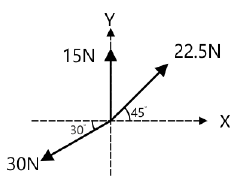4 M
1(b) A smooth circular cylinder of weight W and radius R rest in a V shape groove whose sides are inclined at angles ? & ? to the horizontal as shown. Find the reaction RA and RB at the point of contact.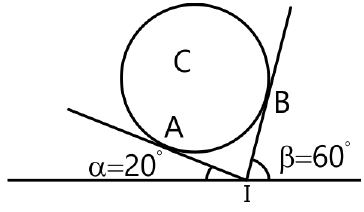4 M
1(c) For the block shown in figure, find the maximum value of P, which will just disturb the equilibrium of the system.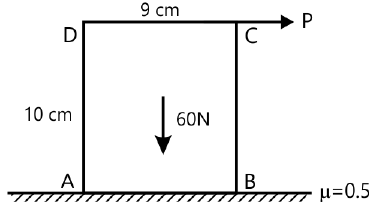4 M
1(d) A particle moving in the positive direction has an acceleration a=100-4v2 m/s2. Determine, the time interval and displacement of a particle when speed changes from 1 m/s to 3 m/s.
4 M
1(e) A vertical lift of total mass 750 kg acquires an upward velocity of 3m/s over a distance of 4m moving with constant acceleration starting from rest. Calculate the tension in the cable.
4 M

2(a) Replace the system of forces and couples by a single force and locate the point on the x-axis through which the line of action of the resultant passes.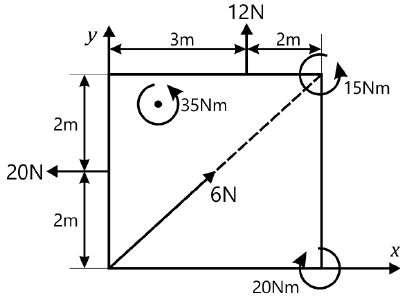6 M
2(b) Two identical rollers each of weight 500 N and radius r are kept on a right angle frame ABC having negligible weight. Assuming smooth surfaces find the reactions induced at all contact surfaces.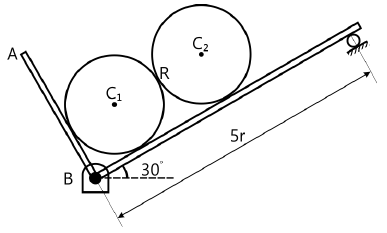8 M
2(c) A body of mass 2 kg is projected upwards from the surfaces of the ground at t=0 with velocity 20 m/s. At the same time another body of mass 2 kg is dropped along the same line from a height of 25m. If they collide elastically, find the velocities of body A and B just after collision.
6 M

3(a) Find the centroid of shaded area.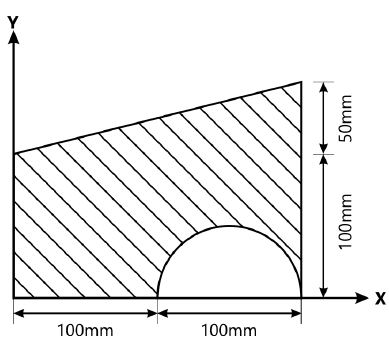8 M
3(b) A rectangular parallelopiped carries 4 forces as shown in figure. Reduce the force system to a resultant force applied at the origin and a moment around origin. OA=5m, OB=2m, OC=4m.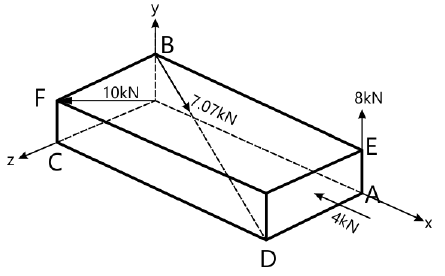6 M
3(c) A spring of stiffness k is placed horizontally and a ball of mass m strikes the spring with a velocity v. Find the maximum compression of the spring. Take m=5 kg, k=500 N/m and v=3 m/s.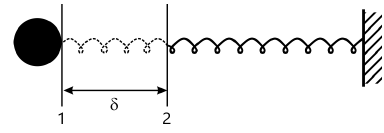6 M

4(a) Find the support reactions for the beam loaded and supported as shown in figure.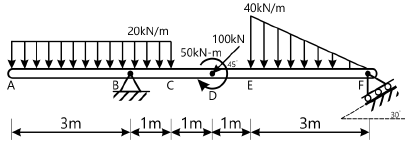8 M
4(b) A ball thrown with speed of 12 m/s at an angle of 600 with a building strikes the ground 11.3 m horizontally from the foot of the building as shown in figure. Determine the height of the building.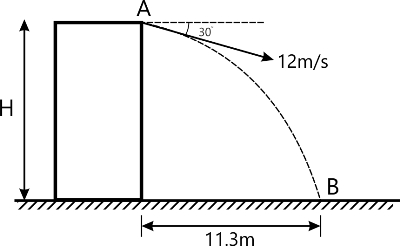6 M
4(c) In a crank and connecting rod mechanism, the length of crank and the connecting rod are 300 mm and 1200 mm respectively. The crank is rotating at 180 rpm. Find the velocity of piston when crank is at 450 with the horizontal.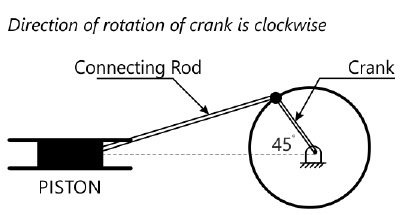6 M

5(a) Referring to truss shown in figure, find:
(i) Reactions at D and C.
(ii) Zero force members.
(iii) Forces in members FE and DC by method of section.
(iv) Forces in other members by method of joints.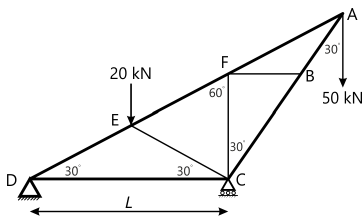8 M
5(b) A point moves along a path y=(x2/3) with a constant speed of 8 m/s. What are the x and y component of its velocity when x=3? What is the acceleration of the point at this instant?
6 M
5(c) At the position show in figure, the crank AB has angular velocity of 3 rad/sec clockwise. Find the velocity of slider C and the point D at the instant shown. AB=100mm.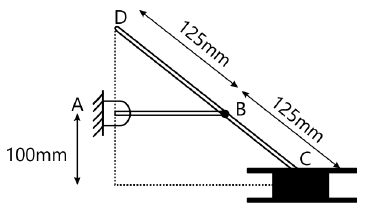6 M

6(a) Force F=80i+50j-60k passes through a point A(6,2,6). Compute its moment about a point B(8,1,4).
4 M
6(b) Assuming the values for ?=0.25 at the floor and 0.3 at the wall and 0.2 between the blocks. Find the minimum value of horizontal force P applied to the lower block that will hold the system in equilibrium.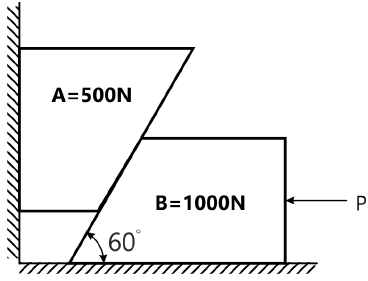8 M
6(c) The car starts from rest and moves in a straight line such that for a short time its velocity is defined by v=(9t2+2t) m/s Where t is in seconds. Determine its position and acceleration when t=3 seconds.
4 M
6(d) Three m1, m2 and m3 of masses 1.5kg, 2kg & 1kg respectively are placed on a rough surface with ?=0.2 as shown. If a force F is applied to accelerate the blocks at 3 m/s2, what will be the force that 1.5 kg block exerts on 2 kg block?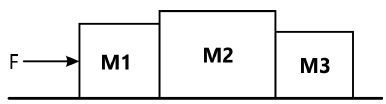4 M

More question papers from Engineering Mechanics